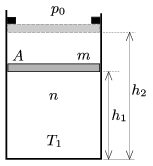Mathematical and Physical Journal
for High Schools
Issued by the MATFUND Foundation
 Already signed up? New to KöMaL?

#Problem P. 4581. (November 2013)

P. 4581. A frictionlessly moveable piston of mass m confines n=0.25 moles of diatomic gas at a temperature of T1=300 K in a vertical cylinder, closed at its bottom, of cross section A=2 dm2. The piston is at a distance of h1=3 dm from the bottom and the ambient pressure is p0=105 Pa.

The gas is heated in two steps. The first step lasts until the piston reaches the stoppers, in this stage the piston is h2=5 dm from the bottom of the cylinder. The added heat in the second step, which begins when the piston reaches the stopper, istimes as much as the added heat in the first step.

a) Determine the mass m of the piston.

b) What is the total force exerted by the stoppers on the piston at the end of the second heating step?

c) Plot the pressure of the gas as a function of the absolute temperature.(5 pont)

Deadline expired on December 10, 2013.

Sorry, the solution is available only in Hungarian. Google translation

Megoldásvázlat. $\displaystyle a)$ A dugattyú tömege: 7,9 kg.

$\displaystyle b)$ $\displaystyle F=1{,}66~$kN.

### Statistics:

 114 students sent a solution. 5 points: 58 students. 4 points: 34 students. 3 points: 14 students. 2 points: 2 students. 1 point: 3 students. Unfair, not evaluated: 3 solutionss.

Problems in Physics of KöMaL, November 2013